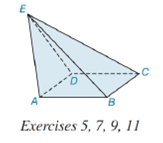Chapter 9.2, Problem 7E### Elementary Geometry for College St...

6th Edition
Daniel C. Alexander + 1 other
ISBN: 9781285195698

#### Solutions

Chapter
Section### Elementary Geometry for College St...

6th Edition
Daniel C. Alexander + 1 other
ISBN: 9781285195698
Textbook Problem
1 views

# Consider the square pyramid in Exercise 5.a) How many vertices does it have?b) How many edges (lateral edges plus base edges) does it have?c) How many faces (lateral faces plus bases) does it have?d) At which point are the lateral edges concurrent?To determine

(a)

To find:

The number of vertices in the given figure.

Explanation

Given:

The figure given below

and base ABCD is a square.

Properties Used:

A pyramid is made by connecting a base to an apex or vertex. The base is flat with straight edges, no curves, hence, a polygon and all other faces are triangles. The altitude/height of the pyramid is the line segment from the vertex perpendicular to the plane of the base

To determine

(b)

To find:

The number of edges in the given figure.

To determine

(c)

To find:

The number of faces in the given figure.

To determine

(d)

To find:

Whether the given figure is a regular hexagonal pyramid.

### Still sussing out bartleby?

Check out a sample textbook solution.

See a sample solution

#### The Solution to Your Study Problems

Bartleby provides explanations to thousands of textbook problems written by our experts, many with advanced degrees!

Get Started